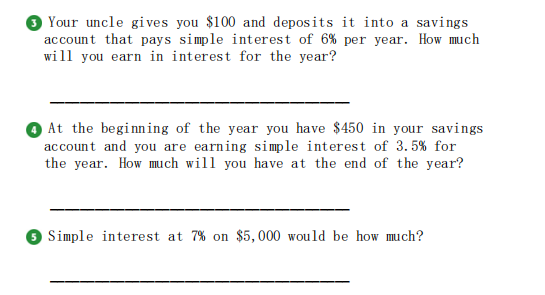### Still have math questions?

Arithmetic
Question3)Your uncle gives you $$\ 100$$ and deposits it into a savings account that pays simple interest of $$6 \%$$ per year. How much will you earn in interest for the year?

(4) At the beginning of the year you have $$\ 450$$ in your savings account and you are earning simple interest of $$3.5 \%$$ for the year. How much will you have at the end of the year?

5) Simple interest at $$7 \%$$ on $$\ 5,000$$ would be how much?

3) $6 4)$ 465.75